Generally, real systems are nonlinear. To design an MPC controller for a nonlinear system, you can model the plant in Simulink®.

Although an MPC controller can regulate a nonlinear plant, the model used within the controller must be linear. In other words, the controller employs a linear approximation of the nonlinear plant. The accuracy of this approximation significantly affects controller performance.

To obtain such a linear approximation, you linearize the nonlinear plant at a specified operating point.

Note

The following examples require Simulink Control Design™ software.

You can linearize a Simulink model:

### Linearization Using MATLAB Code

This example shows how to obtain a linear model of a plant using a MATLAB script.

For this example the CSTR model, `CSTR_OpenLoop`, is linearized. The model inputs are the coolant temperature (manipulated variable of the MPC controller), limiting reactant concentration in the feed stream, and feed temperature. The model states are the temperature and concentration of the limiting reactant in the product stream. Both states are measured and used for feedback control.

The operating point defines the nominal conditions at which you linearize a model. It is usually a steady-state condition.

Suppose that you plan to operate the CSTR with the output concentration, `C_A`, at $2\phantom{\rule{0.2777777777777778em}{0ex}}kmol/{m}^{3}$. The nominal feed concentration is $10\phantom{\rule{0.2777777777777778em}{0ex}}kmol/{m}^{3}$, and the nominal feed temperature is 300 K.

Create and visualize an operating point specification object to define the steady-state conditions.

```opspec = operspec('CSTR_OpenLoop'); opspec = addoutputspec(opspec,'CSTR_OpenLoop/CSTR',2); opspec.Outputs(1).Known = true; opspec.Outputs(1).y = 2; opspec```
```opspec = Operating point specification for the Model CSTR_OpenLoop. (Time-Varying Components Evaluated at time t=0) States: ---------- x Known SteadyState Min Max dxMin dxMax ___________ ___________ ___________ ___________ ___________ ___________ ___________ (1.) CSTR_OpenLoop/CSTR/C_A 8.5695 false true 0 Inf -Inf Inf (2.) CSTR_OpenLoop/CSTR/T_K 311.267 false true 0 Inf -Inf Inf Inputs: ---------- u Known Min Max _____ _____ _____ _____ (1.) CSTR_OpenLoop/Coolant Temperature 0 false -Inf Inf Outputs: ---------- y Known Min Max _____ _____ _____ _____ (1.) CSTR_OpenLoop/CSTR 2 true -Inf Inf ```

Search for an operating point that satisfies the specifications.

`op1 = findop('CSTR_OpenLoop',opspec);`
``` Operating point search report: --------------------------------- ```
```opreport = Operating point search report for the Model CSTR_OpenLoop. (Time-Varying Components Evaluated at time t=0) Operating point specifications were successfully met. States: ---------- Min x Max dxMin dx dxMax ___________ ___________ ___________ ___________ ___________ ___________ (1.) CSTR_OpenLoop/CSTR/C_A 0 2 Inf 0 -4.6683e-12 0 (2.) CSTR_OpenLoop/CSTR/T_K 0 373.1311 Inf 0 5.5451e-11 0 Inputs: ---------- Min u Max ________ ________ ________ (1.) CSTR_OpenLoop/Coolant Temperature -Inf 299.0349 Inf Outputs: ---------- Min y Max ___ ___ ___ (1.) CSTR_OpenLoop/CSTR 2 2 2 ```

The calculated operating point is `C_A` = $2\phantom{\rule{0.2777777777777778em}{0ex}}kmol/{m}^{3}$ and `T_K` = 373 K. Notice that the steady-state coolant temperature is also given as 299 K, which is the nominal value of the input used to control the plant.

To specify:

• Values of known inputs, use the `Input.Known` and `Input.u` fields of `opspec`

• Initial guesses for state values, use the `State.x` field of `opspec`

For example, the following code specifies the coolant temperature as 305 K and initial guess values of the `C_A` and `T_K` states before calculating the steady-state operating point:

```opspec = operspec('CSTR_OpenLoop'); opspec.States(1).x = 1; opspec.States(2).x = 400; opspec.Inputs(1).Known = true; opspec.Inputs(1).u = 305; op2 = findop('CSTR_OpenLoop',opspec)```
``` Operating point search report: --------------------------------- ```
```opreport = Operating point search report for the Model CSTR_OpenLoop. (Time-Varying Components Evaluated at time t=0) Operating point specifications were successfully met. States: ---------- Min x Max dxMin dx dxMax ___________ ___________ ___________ ___________ ___________ ___________ (1.) CSTR_OpenLoop/CSTR/C_A 0 1.7787 Inf 0 -1.5987e-14 0 (2.) CSTR_OpenLoop/CSTR/T_K 0 376.5371 Inf 0 7.1054e-14 0 Inputs: ---------- Min u Max ___ ___ ___ (1.) CSTR_OpenLoop/Coolant Temperature 305 305 305 Outputs: None ---------- ```
```op2 = Operating point for the Model CSTR_OpenLoop. (Time-Varying Components Evaluated at time t=0) States: ---------- x ________ (1.) CSTR_OpenLoop/CSTR/C_A 1.7787 (2.) CSTR_OpenLoop/CSTR/T_K 376.5371 Inputs: ---------- u ___ (1.) CSTR_OpenLoop/Coolant Temperature 305 ```

Specify Linearization Inputs and Outputs

If the linearization input and output signals are already defined in the model, as in `CSTR_OpenLoop`, then use the following to obtain the signal set.

`io = getlinio('CSTR_OpenLoop');`

Otherwise, specify the input and output signals as shown here.

```io(1) = linio('CSTR_OpenLoop/Coolant Temperature',1,'input'); io(2) = linio('CSTR_OpenLoop/Feed Concentration',1,'input'); io(3) = linio('CSTR_OpenLoop/Feed Temperature',1,'input'); io(4) = linio('CSTR_OpenLoop/CSTR',1,'output'); io(5) = linio('CSTR_OpenLoop/CSTR',2,'output');```

Linearize Model

Linearize the model using the specified operating point, `op1`, and input/output signals, `io`.

`sys = linearize('CSTR_OpenLoop',op1,io)`
```sys = A = C_A T_K C_A -5 -0.3427 T_K 47.68 2.785 B = Coolant Temp Feed Concent Feed Tempera C_A 0 1 0 T_K 0.3 0 1 C = C_A T_K CSTR/1 0 1 CSTR/2 1 0 D = Coolant Temp Feed Concent Feed Tempera CSTR/1 0 0 0 CSTR/2 0 0 0 Continuous-time state-space model. ```

Linearize the model also around the operating point, `op2`, using the same input/output signals.

`sys = linearize('CSTR_OpenLoop',op2,io)`
```sys = A = C_A T_K C_A -5.622 -0.3458 T_K 55.1 2.822 B = Coolant Temp Feed Concent Feed Tempera C_A 0 1 0 T_K 0.3 0 1 C = C_A T_K CSTR/1 0 1 CSTR/2 1 0 D = Coolant Temp Feed Concent Feed Tempera CSTR/1 0 0 0 CSTR/2 0 0 0 Continuous-time state-space model. ```

### Linearization Using Model Linearizer in Simulink Control Design

This example shows how to linearize a Simulink model using the Model Linearizer, provided by the Simulink Control Design software.

This example uses the CSTR model, `CSTR_OpenLoop`.

`open_system('CSTR_OpenLoop')`

Specify Linearization Inputs and Outputs

The linearization inputs and outputs are already specified for `CSTR_OpenLoop`. The input signals correspond to the outputs from the `Feed Concentration`, `Feed Temperature`, and `Coolant Temperature` blocks. The output signals are the inputs to the `CSTR Temperature` and `Residual Concentration` blocks.

To specify a signal as a linearization input or output, first in the Simulink Apps tab, click Linearization Manager. Then, in the Simulink model window, click the signal. Finally, in the Insert Analysis Points gallery, in the Closed Loop section, select either Input Perturbation for a linearization input or Output Measurement for a linearization output.

Open Model Linearizer

To open the Model Linearizer, in the tab, click Model Linearizer.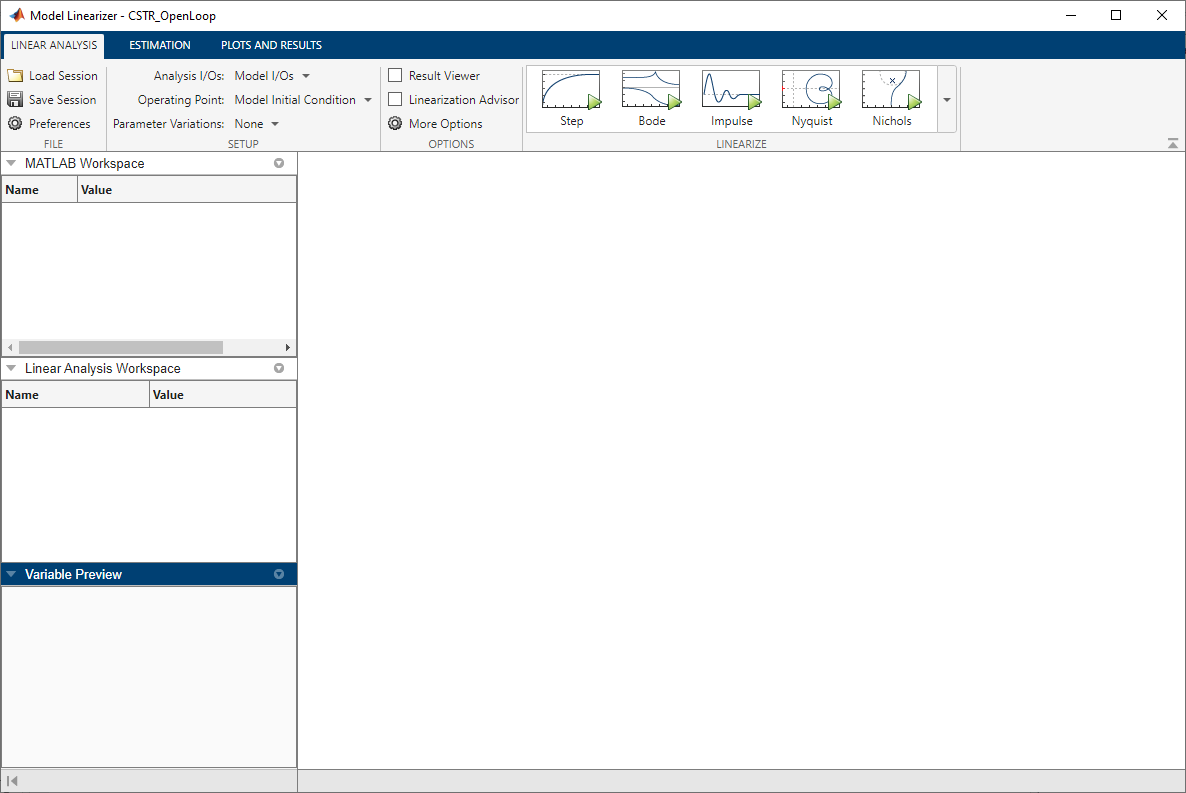Specify Residual Concentration as Known Trim Constraint

To specify the residual concentration as a known trim constant, first in the Simulink Apps tab, click Linearization Manager. Then, in the Simulink model window, click the `CA` output signal from the `CSTR` block. Finally, in the Insert Analysis Points gallery, in the Trim section, select Trim Output Constraint.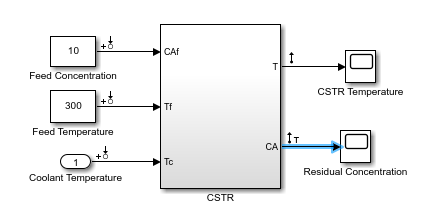In the Model Linearizer, on the Linear Analysis tab, select Operating Point > Trim Model.

In the Trim the model dialog box, on the Outputs tab:

• Select the Known check box for ```Channel - 1``` under CSTR_OpenLoop/CSTR.

• Set the corresponding Value to `2` kmol/m3.Create and Verify Operating Point

In the Trim the model dialog box, click .

The Trim progress viewer window opens up showing the optimization progress towards finding a point in the state-input space of the model with the characteristics specified in the States, Inputs, and Outputs tabs. After the optimization process terminates, close the trim progress window as well as the Trim the model dialog box.

The operating point `op_trim1` displays in the Linear Analysis Workspace of Model Linearizer. Select `op_trim1` to display basic information in the Linear Analysis Workspace section.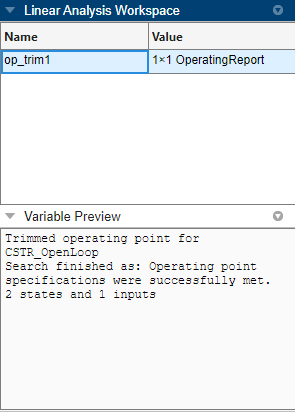Double click `op_trim1` to view the resulting operating point in the Edit dialog box.

In the Edit dialog box, select the Input tab.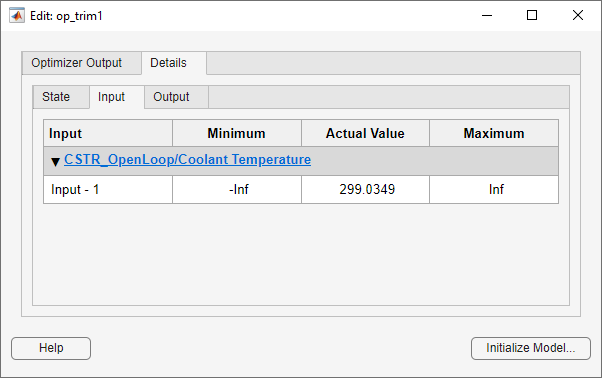The coolant temperature at steady state is 299 K, as desired. Close the Edit dialog box.

Linearize Model

On the Linear Analysis tab, in the Operating Point drop-down list, make sure `op_trim1` is selected.

In the section, clickto linearize the Simulink model and display the step response of the linearized model.

This option creates the linear model `linsys1` in the Linear Analysis Workspace and generates a step response for this model. `linsys1` uses `op_trim1` as its operating point.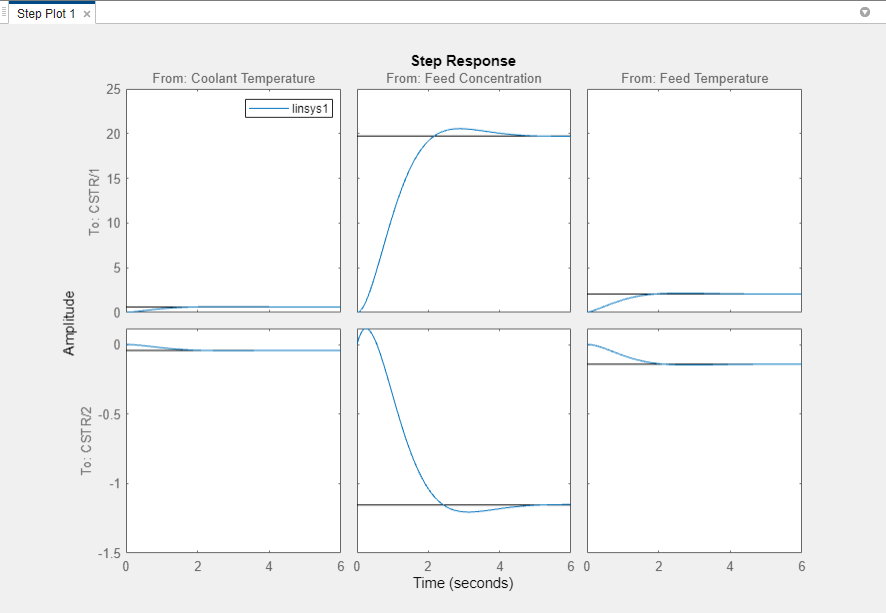The step response from feed concentration to output `CSTR/2` displays an interesting inverse response. An examination of the linear model shows that `CSTR/2` is the residual CSTR concentration, `C_A`. When the feed concentration increases, `C_A` increases initially because more reactant is entering, which increases the reaction rate. This rate increase results in a higher reactor temperature (output `CSTR/1`), which further increases the reaction rate and `C_A` decreases dramatically.

Export Linearization Result

If necessary, you can repeat any of these steps to improve your model performance. Once you are satisfied with your linearization result, in the Model Linearizer, drag the linear model from the Linear Analysis Workspace section of Model Linearizer to the MATLAB Workspace section just above it. You can now use your linear model to design an MPC controller.